Courses

# Doc: Kinetic Energy and Potential Energy Class 11 Notes | EduRev

## Class 11 : Doc: Kinetic Energy and Potential Energy Class 11 Notes | EduRev

The document Doc: Kinetic Energy and Potential Energy Class 11 Notes | EduRev is a part of the Class 11 Course Physics Class 11.
All you need of Class 11 at this link: Class 11

9. ENERGY

A body is said to possess energy if it has the capacity to do work. When a body possessing energy does some work, part of its energy is used up. Conversely if some work is done upon an object, the object will be given some energy. Energy and work are mutually convertiable.

There are various forms of energy. Heat, electricity, light, sound and chemical energy are all familiar forms. In studying mechanics, we are however concerned chiefly with mechanical energy. This type of energy is a property of movement or position.

9.1 kinetic Energy

Kinetic energy (K.E.), is the capacity of a body to do work by virtue of its motion.

If a body of mass m has velocity v its kinetic energy is equivalent to the work, which an external force would have to do to bring the body from rest up to its velocity v.

The numerical value of the kinetic energy can be calculated from the formula

K.E. =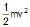...(8)

Since both m and v2 are always positive, K.E. is always positive and does not depend upon the direction of motion of the body.

9.2 Potential Energy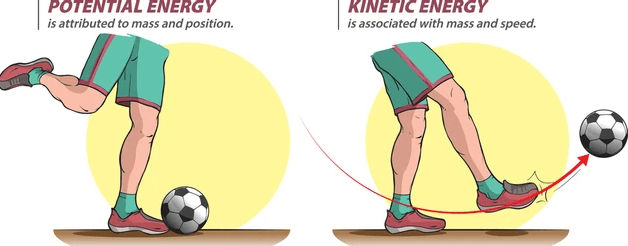Potential energy is energy of the body by virtue of its position. A body is capable to do work by virtue of its position, configuration or state of strain.

Now relation between Potential energy and work done is

W.D = - DU

where DU is change in potential energy

There are two common forms of potential energy, gravitational and elastic.

Important points related to Potential energy :

1. Potential energy is a straight function (defined only for position)

2. Potential energy of a point depends on a reference point

3. Potential energy difference between two position doesn't depend on the frame of reference.

4. Potential energy is defined only for conservative force because work done by conservative force is path independent.

5. If we define Potential energy for non conservative force then we have to define P.E. of a single point through different path which gives different value of P.E. at single point that doesn't make any sense.

9.2.1 (a) Gravitational Potential Energy :

It is possessed by virtue of height.

When an object is allowed to fall from one level to a lower level it gains speed due to gravitational pull, i.e., it gains kinetic energy. Therefore, in possessing height, a body has the ability to convert its gravitational potential energy into kinetic energy.

The gravitational potential energy is equivalent to the negative of the amount of work done by the weight of the body in causing the descent.

If a mass m is at a height h above a lower level the P.E. possessed by the mass is (mg) (h).

Since h is the height of an object above a specified level, an object below the specified level has negative potential energy.

Therefore GPE = ± mgh ...(9)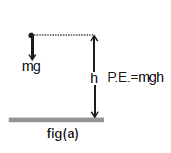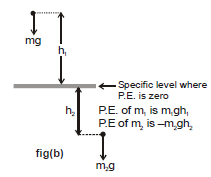• The chosen level from which height is measured has no absolute position. It is important therefore to indicate clearly the zero P.E. level in any problem in which P.E. is to be calculated.
• GPE = ± mgh is applicable only when h is very small in comparison to the radius of the earth. We have discussed GPE in detail in 'GRAVITATION'.

9.2.2 (b) Elastic Potential Energy : It is a property of stretched or compressed springs.

The end of a stretched elastic spring will begin to move if it is released. The spring. therefore possesses potential energy due to its elasticity. (i.e., due to change in its configuration)

The amount of elastic potential energy stored in a spring of natural length a and spring constant k when it is extended by a length x (from the natural length) is equivalent to the amount of work necessary to produce the extension.

Elastic Potential Energy =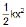...(10)

It is never negative whether the spring is extended or compressed.

Proof :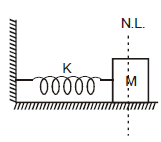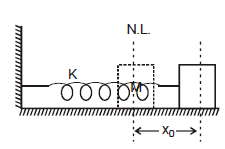Consider a spring block system as shown in the figure and let us calculate work done by spring when the block is displaceed by xfrom the natural length.

At any moment if the elongation in spring is x, then the force on the block by the spring is kx towards left. Therefore, the work done by the spring when block further displaces by dx

dW = - kx dx

Total work done by the spring, W = -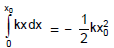Similarly, work done by the spring when it is given a compression x0 is -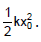.

•  : We assume zero potential energy at natural length of the spring :

10. Conservative force and potential energy :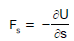i.e. the projection of the force field , the vector F, at a given point in the direction of the displacement r equals the derivative of the potential energy U with respect to a given direction, taken with the opposite sign. The designation of a partial derivative ∂/∂ s emphasizes the fact of deriving with respect to a definite direction.

So, having reversed the sign of the partial derivatives of the function U with respect to x, y, z, we obtain the projection Fx, Fy and Fz of the vector F on the unit vectors i, j and k. Hence, one can readily find the vector itself :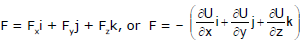The quantity in parentheses is referred to as the scalar gradient of the function U and is denoted by grad U or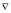U. We shall use the second, more convenient, designation where ("nabla") signifies the symbolic vector or operator=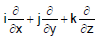Potential Energy curve :

A graph plotted between the PE of a particle and its displacement from the center of force field is called PE curve.

Using graph, we can predict the rate of motion of a particle at various positions.

Force on the particle is F(x) =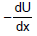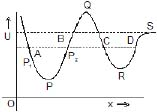Case : I On increasing x, if U increases, force is in (-) ve x direction i.e. attraction force.

Case : II On increasing x, if U decreases, force is in ( + ) ve x-direction i.e. repulsion force.

Different positions of a particle :

Position of equilibrium

If net force acting on a body is zero, it is said to be in equilibrium. For equilibrium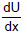= 0. Points P, Q, R and S are the states of equilibrium positions.

Types of equilibrium :

• Stable equilibrium :

When a particle is displaced slightly from a position and a force acting on it brings it back to the             initial position, it is said to be in stable equilibrium position.

Necessary conditions: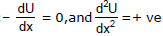In figure P and R point shows stable equilibrium point.

Unstable Equilibrium :

When a particle is displaced slightly from a position and force acting on it tries to displace the particle further away from the equilibrium position, it is said to be in unstable equilibrium.

Condition :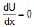potential energy is maximum i.e. =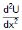= - ve

Q point in figure shows unstable equilibrium point

Neutral equilibrium :

In the neutral equilibrium potential energy is constant. When a particle is displaced from its position it does not experience any force acting on it and continues to be in equilibrium in the displaced position. This is said to be neutral equilibrium.

In figure S is the neutral point

Condition :,= 0

Ex.12 The potential energy between two atoms in a molecule is given by, U(x)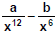, where a and b are positive constants and x is the distance between the atoms. The system is in stable equilibrium when -

(A) x = 0

(B) x =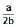(C) x =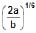(D) x =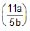Sol. (C)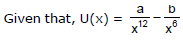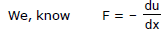= (-12) a x -13 -(-6b) x-7 = 0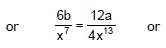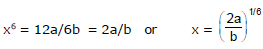Ex.13 The potential energy of a conservative system is given by U = ax2 - bx where a and b are positive constants. Find the equilibrium position and discuss whether the equilibrium is stable, unstable or neutral.

Sol.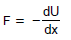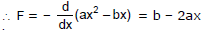For equilibrium F = 0 or b - 2ax = 0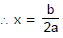From the given equation we can see that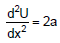(positive), i.e., U is minimum.

Therefore,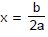is the stable equilibrium position,

Offer running on EduRev: Apply code STAYHOME200 to get INR 200 off on our premium plan EduRev Infinity!

## Physics Class 11

75 videos|312 docs|152 tests

,

,

,

,

,

,

,

,

,

,

,

,

,

,

,

,

,

,

,

,

,

;